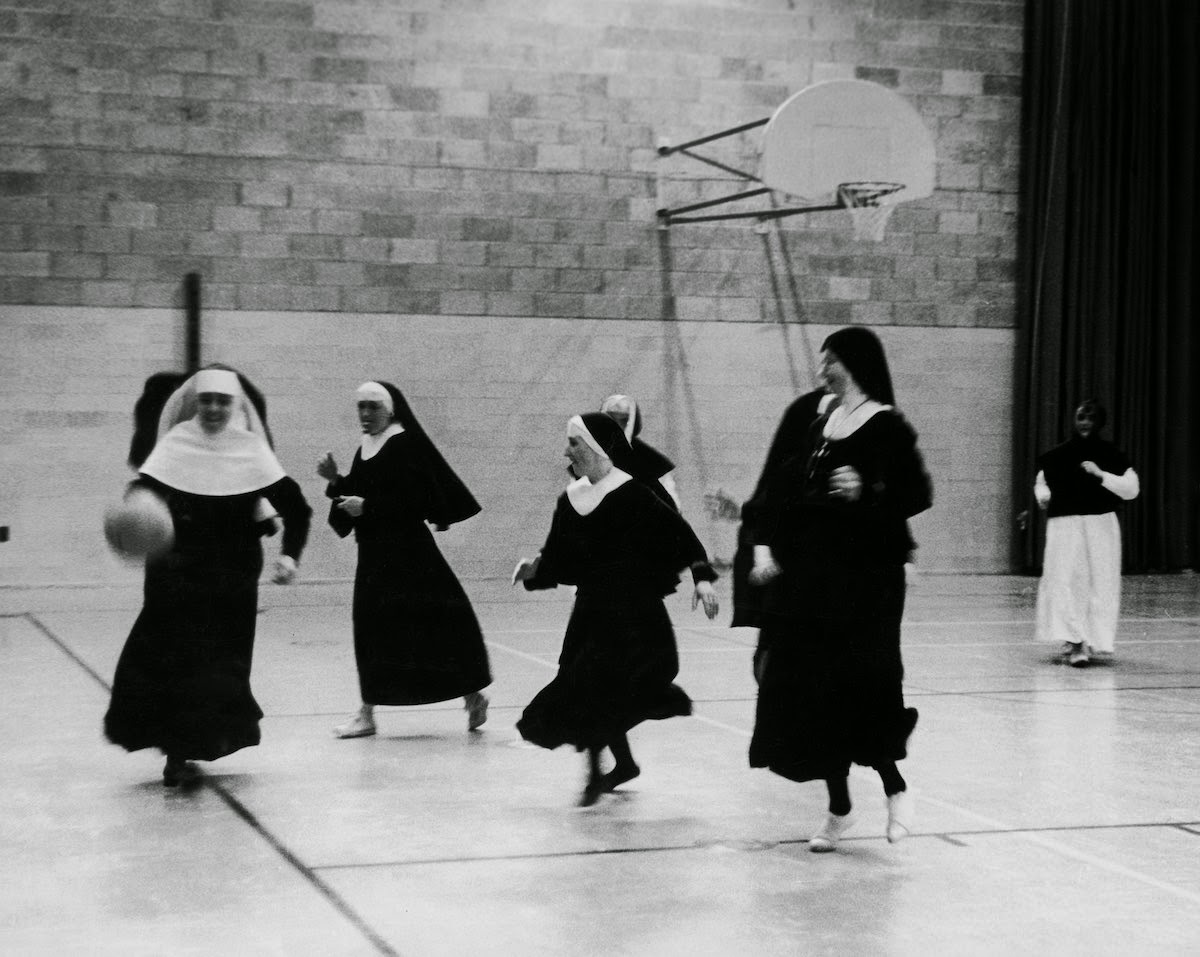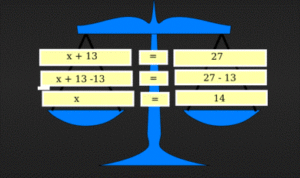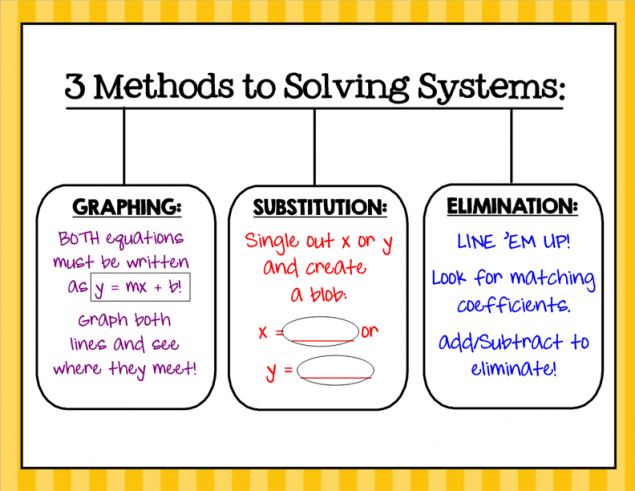## MS Math Jan 31 – Feb 4, 2022Happy Catholic Schools Week!

We are grateful to be part of a long history of Catholic education!

6th Grade Math – Mrs. Evans

This week we will finish our study of unit conversions with a Chapter 6 test on Thursday.  In this chapter we have learned that units can help us to set up and solve problems.  Just like numbers, units can “cancel out” leaving the correct units if we set up our problem correctly.  Our test will also cover using rate, distance and time formulas.  While rate, distance and time are related in one way, we can rearrange the formula to solve for whatever of the three we are needing.  Here are the formulas in different forms:Math Grade Math – Mrs. Evans and Mrs. VonFeldtThis week we will conclude our algebra studies with a review on Monday and a test on Wednesday.  This topic is cumulative as each skill builds the foundation for the next.  It will cover expressions, equations and inequalities.  For students wanting to practice this week, IXL.COM (sections R, T and U) would be an excellent place to do so.  It provides immediate feedback and, when you scroll to the bottom of the page, explanation and examples of problems done correctly.  If you want more help, please reach out to Mrs. Evans or Mrs. VonFeldt.   Later in the week, we will begin our study of geometry with scale drawings.  Here is a link to IXL.         IXL | Learn 7th grade math

8th Grade Math – Mrs. Ernest

The 8th graders will be jumping into Chapter 5, which explores angles, triangles, and similarity! Students will learn how to use a protractor and learn the following terms:Algebra – Mrs. VonFeldt

This week, we are exploring what happens when you have a set of two equations graphed on the same axes. These are called systems of linear equations and the solution is the ordered pair where the two lines intersect, or a point that is on both lines. We will be learning three methods for solving systems of equations: Graphing, Substitution, and Elimination. Each method can be useful depending on how the equations are written. See the image below for a brief explanation of each strategy.## MS Math – Jan 24-286th Grade Math – Mrs. Evans

This week we will continue to learn about converting between units of measurement.  We will be working with both customary units and metric units.  By using conversion factors, we can convert between any two units regardless of the system.  Here is how we can use a conversion factor:The metric system is a base ten system so you can also make conversions with this method:Math Grade Math – Mrs. Evans and Mrs. VonFeldt

This week we will continue our study of algebra as we write, model and solve one and two step inequalities.  While the solution of an equation is a single value, the solution of an inequality is a range of numbers.  With inequalities, we can use the same logic as solving equations.  The DIFFERENCE between solving equations and inequalities, is that FOR INEQUALITIES, if you multiply or divide both sides by a negative number, you must reverse the inequality symbol for it to remain true.  Here are summaries on how to solve equations and inequalities:8th Grade Math – Mrs. Ernest

Last week, 8th graders perfected their ability to find whether or not fractions were proportional to one another using cross multiplication. This week, we will extend that learning by finding similar figures, solving for missing side lengths, and building our confidence with geometric word problems and graphing.

IXL review:
Level 1:
Level 2:
Level 3:
Unit Rates Bonus Practice:

Algebra – Mrs. VonFeldt

This week, we finished Chapter 5 and our study of inequalities. Students are now familiar with graphing inequalities in one or two variables. We will continue to practice solving and graphing equations as we begin to look at different ways to solve systems of equations.

© 2023 Assumption Catholic School | 2116 Cornwall Ave, Bellingham, WA 98225
Phone: 360.733.6133 | Fax: 360.647.4372
Email: theoffice@school.assumption.org
Dashboard | Web design and development by Olywebdev.com